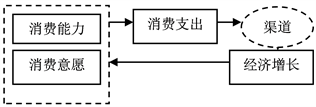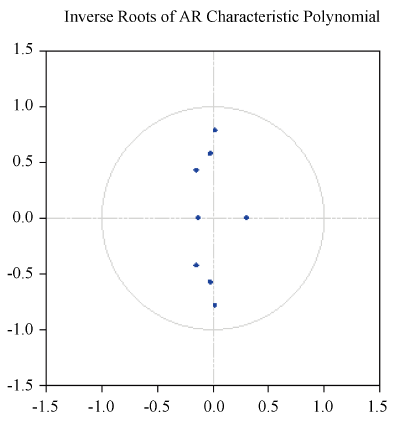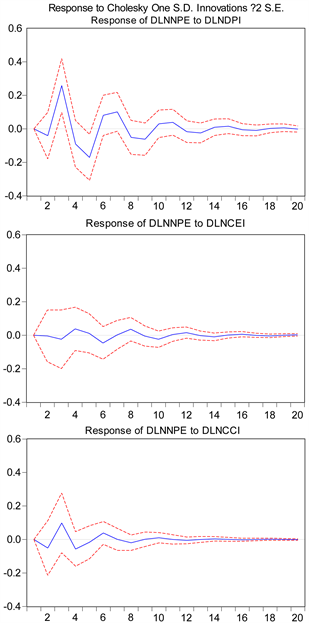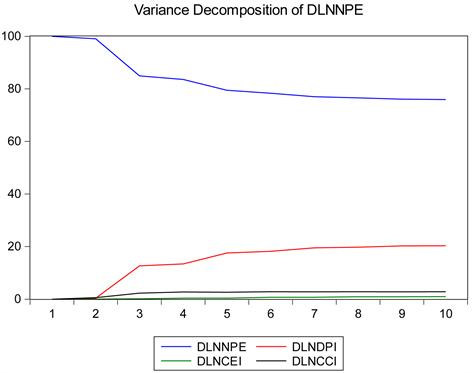消费意愿和能力对消费支出影响的实证分析 An Empirical Analysis of the Influence of Consumer Willingness and Ability on Consumer Expenditure

• 全文下载: PDF(924KB)    PP.18-25   DOI: 10.12677/SA.2019.81003
• 下载量: 128  浏览量: 389

Consumer spending is not only affected by the macroeconomic situation, but also closely related to consumers’ willingness to consume (confidence, expectations, etc.) and the ability to consume. In this paper, the consumer confidence index CCI and the consumer expectation index CEI are used as indicators to measure consumer confidence. The per capita disposable income DPI of urban residents is used as an indicator to measure consumption capacity, while the consumer expenditure is based on the per capita consumption expenditure NPE of urban residents. The VAR model was established by adopting co-integration analysis and impulse response methods, and empirically analyzed the influence of consumer confidence and consumption ability on consumer expenditure. The results show that CEI has a significant impact on NPE, and when analyzing the relationship between all variables, it is found that the contribution rate of DPI to NPE is the largest.

1. 引言

2. 模型选择与变量选取Figure 1. Mechanism of “Conduction-Feedback”

(一) 指标与数据选取Figure 2. Variation trend of each index after logarithm

(二) 模型设定

VAR (Vector Auto Regressive)模型由Sims (1980)提出，是当今世界上分析经济系统动态性的主流模型之一。VAR模型可以表述如下：

${Y}_{t}={\alpha }_{1}{Y}_{t-1}+{\alpha }_{2}{Y}_{t-2}+\cdots +{\alpha }_{p}{Y}_{t-p}+{\epsilon }_{t}$ (1)

3. 实证分析

(一) 稳定性检验及协整分析Table 2. Cointegration testing process

(二) VAR模型及脉冲响应分析

1) AR根检验结果Figure 3. AR root test results

2) VAR模型估计

$\begin{array}{l}\text{dlnnpe}=0.0\text{34}-0.\text{65}0\text{dlnnpe}\left(-\text{1}\right)-0.\text{382dlnnpe}\left(-\text{2}\right)\\ \text{\hspace{0.17em}}\text{\hspace{0.17em}}\text{\hspace{0.17em}}\text{\hspace{0.17em}}\text{\hspace{0.17em}}\text{\hspace{0.17em}}\text{\hspace{0.17em}}\text{\hspace{0.17em}}\text{\hspace{0.17em}}\text{\hspace{0.17em}}\text{\hspace{0.17em}}\left(\text{5}.\text{359}\right)\text{\hspace{0.17em}}\text{\hspace{0.17em}}\text{\hspace{0.17em}}\text{\hspace{0.17em}}\text{\hspace{0.17em}}\text{\hspace{0.17em}}\text{\hspace{0.17em}}\text{\hspace{0.17em}}\text{\hspace{0.17em}}\text{\hspace{0.17em}}\text{\hspace{0.17em}}\text{\hspace{0.17em}}\text{\hspace{0.17em}}\left(-\text{8}.\text{871}\right)\text{\hspace{0.17em}}\text{\hspace{0.17em}}\text{\hspace{0.17em}}\text{\hspace{0.17em}}\text{\hspace{0.17em}}\text{\hspace{0.17em}}\text{\hspace{0.17em}}\text{\hspace{0.17em}}\text{\hspace{0.17em}}\text{\hspace{0.17em}}\text{\hspace{0.17em}}\text{\hspace{0.17em}}\text{\hspace{0.17em}}\left(-\text{5}.\text{224}\right)\\ \text{\hspace{0.17em}}\text{\hspace{0.17em}}\text{\hspace{0.17em}}\text{\hspace{0.17em}}\text{\hspace{0.17em}}\text{\hspace{0.17em}}\text{\hspace{0.17em}}\text{\hspace{0.17em}}\text{\hspace{0.17em}}\text{\hspace{0.17em}}\text{\hspace{0.17em}}\text{\hspace{0.17em}}+\text{1}.\text{183dlncei}-\text{1}.0\text{9}0\text{dlncci}+0.\text{445dlndpi}\\ \text{\hspace{0.17em}}\text{\hspace{0.17em}}\text{\hspace{0.17em}}\text{\hspace{0.17em}}\text{\hspace{0.17em}}\text{\hspace{0.17em}}\text{\hspace{0.17em}}\text{\hspace{0.17em}}\text{\hspace{0.17em}}\text{\hspace{0.17em}}\text{\hspace{0.17em}}\text{\hspace{0.17em}}\text{\hspace{0.17em}}\text{\hspace{0.17em}}\text{\hspace{0.17em}}\text{\hspace{0.17em}}\text{\hspace{0.17em}}\text{\hspace{0.17em}}\text{\hspace{0.17em}}\text{\hspace{0.17em}}\left(\text{1}.\text{474}\right)\text{\hspace{0.17em}}\text{\hspace{0.17em}}\text{\hspace{0.17em}}\text{\hspace{0.17em}}\text{\hspace{0.17em}}\text{\hspace{0.17em}}\text{\hspace{0.17em}}\text{\hspace{0.17em}}\left(-\text{1}.\text{191}\right)\text{\hspace{0.17em}}\text{\hspace{0.17em}}\text{\hspace{0.17em}}\text{\hspace{0.17em}}\text{\hspace{0.17em}}\text{\hspace{0.17em}}\text{\hspace{0.17em}}\text{\hspace{0.17em}}\text{\hspace{0.17em}}\left(\text{1}0.\text{7}0\text{8}\right)\end{array}$ (2)

$\begin{array}{l}{Y}_{t}=\left[\begin{array}{cccc}-0.361& -0.282& 0.126& -0.502\\ -0.023& -0.667& 0.818& -0.050\\ -0.014& -0.796& 0.944& -0.038\\ 0.800& -0.379& 0.523& -1.129\end{array}\right]×{Y}_{t-1}\\ \text{\hspace{0.17em}}\text{\hspace{0.17em}}\text{\hspace{0.17em}}\text{\hspace{0.17em}}\text{\hspace{0.17em}}+\left[\begin{array}{cccc}0.278& 0.563& -0.824& -0.428\\ 0.089& 0.356& -0.762& -0.084\\ 0.069& 0.311& -0.637& -0.072\\ 1.340& 1.047& -1.098& -0.785\end{array}\right]×{Y}_{t-2}\\ \text{\hspace{0.17em}}\text{\hspace{0.17em}}\text{\hspace{0.17em}}\text{\hspace{0.17em}}\text{\hspace{0.17em}}+\left[\begin{array}{c}0.049\\ 0.004\\ 0.003\\ 0.026\end{array}\right]+{\epsilon }_{t}\end{array}$ (3)

(三) NPE对各经济指标的脉冲响应分析Figure 4. Impulse response analysis

(四) 方差分解结果Figure 5. Variance decomposition result of dlnnpe

4. 结论

  李成. 消费者信心能预测产出波动吗——来自中国的经验证据[J]. 经济学动态, 2016(12): 88-97.  王岱, 程灵沛, 祝伟. 我国消费者信心的影响因素分析[J]. 宏观经济研究, 2016(4): 48-61.  乔治•阿克洛夫, 罗伯特•席勒. 动物精神[M]. 黄志强, 等, 译. 北京: 中信出版社, 2016: 87-108.  陈彦斌, 唐诗磊. 信心、动物精神与中国宏观经济波动[J]. 金融研究, 2009(9): 89-109.  张道德, 俞林. CPI、PMI与消费者信心指数(CCI)的关系研究及实证分析[J]. 中国物价, 2009(9): 8-11.  李跃辉, 彭嘉莹. 基于VAR-VEC模型的物价指数关系研究[J]. 统计与决策, 2012(15): 19-22.  孙颖. 消费者信心指数与居住类居民消费价格指数相关性研究[J]. 价格理论与实践, 2016(4): 95-97.  邱洋冬. 我国CPI、PMI与消费者信心指数CCI的关系研究[J]. 武汉商学院学报, 2017, 31(1): 44-47.  任韬, 阮敬. 中国消费者信心影响因素实证分析[J]. 统计与信息论坛, 2010, 25(1): 87-90.  陈红, 郭丹, 张佳睿. 货币政策传导信心渠道研究[J]. 当代经济研究, 2015(12): 67-75.  赵好, 袁泽鹏, 赵一琳. 中国消费者信心指数影响因素分析[J]. 合作经济与科技, 2017(16): 78-79.